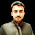# Programming Videos

## Search

### Find Sum of First n Odd Numbers in C, C++

Write a C, C++ Program to find sum of first n odd numbers.

There are multiple approach to solve this problem. Here i use mathematical trick to solve this problem in efficient way.

An odd number is a number which is not a multiple of two. For example - 1 , 3 , 5 etc.

Program to check Even or Odd number
.

Print Even Numbers between 1 to 100.

## How to Calculate Sum of First n Odd Numbers

Let's say we have to calculate the sum of first 10 odd numbers.

First 10 odd numbers are

1 , 3, 5, 7, 9, 11, 13, 15, 17, 19

Check the sequence of first 10 odd numbers it's an arithmetic progression with common difference 2. The formula of arithmetic progression is

So we can conclude that the sum of first n odd numbers is square of n.

## C Program to Find Sum of first n odd numbers

```#include <stdio.h>

int main(void) {

int n,sum;

printf("Enter the number");
scanf("%d",&n);

sum = n*n;

printf("Sum of first %d odd numbers are %d",n,sum);

return 0;
}

```

Output :

Enter the number : 20

Sum of first 20 odd numbers are 400

## Find Sum of First 100 Odd Numbers

METHOD 1:

```#include <iostream>
using namespace std;

int main() {

int i=0, num=0, sum=0;

while (i < 100)
{
/* Check if it's not divisible of 2 */

if(num%2 != 0)
{
/* Add number and increment the value of i */

sum = sum + num;
i++;
}

num++;
}

cout << "The sum of first 100 odd numbers is " << sum;

return 0;
}
```

Output :

The sum of first 100 odd numbers is : 10000

METHOD 2:

We already proved that sum of first n odd numbers is square of n. Using this approach we can directly print the square of n where n is 100.

Find cube root of a number.

Reverse a string using stack.

1.2.